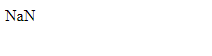# Math.log2() Method with Example in JavaScript

JavaScript | Math.log2() Method: Here, we are going to learn about the log2() method of Math class in JavaScript with Examples.
Submitted by Shivang Yadav, on November 30, 2019

## JavaScript | Math.log2() Method

Math operations in JavaScript are handled using functions of math library in JavaScript. In this tutorial on Math.log2() method, we will learn about the log2() method and its working with examples.

JavaScript has multiple methods in its math library to support mathematical operations in JavaScript.

Math.log2() method in JavaScript is inbuilt. This function returns the value of the logarithmic value of base 2 of the number.

Syntax:

```    Math.log2(n);
```

Parameter(s):

• n – It takes only one value which is the number whose logarithm is to be found.

Return value:

The return type of this method is number, it returns the log2 of the given number.

Example 1: finding the log of an integer.

```<!DOCTYPE html>
<html>

<title>Page Title</title>

<body>
<script>
document.write(Math.log2(3) + "<br>");
document.write(Math.log2(87) + "<br>");
document.write(Math.log2(102) + "<br>");
document.write(Math.log2(512));
</script>
</body>

</html>
```

OutputExample 2: finding log of a string.

```<!DOCTYPE html>
<html>

<title>Page Title</title>

<body>

<script>
document.write(Math.log2("Include help"));
</script>

</body>

</html>
```

OutputWhen a string is passed to the log2() method. It returns NaN denoting the passed value is not a number.

Example 3: Find the log of a complex number.

```<!DOCTYPE html>
<html>

<title>Page Title</title>

<body>

<script>
document.write(Math.log2(1 + 2 i));
</script>

</body>

</html>
```

Output

```Invalid or unexpected token
```

Preparation

What's New

Top Interview Coding Problems/Challenges!